# Median of an Even Set of Numbers

Median of an Even Set of Numbers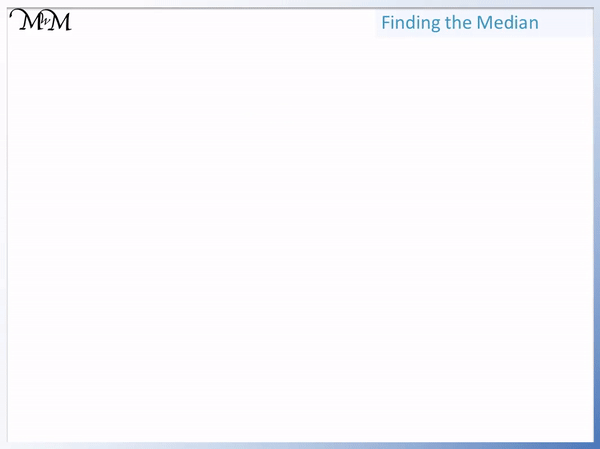• To find the median, put the numbers in order and then find the number in the middle.
• First we arrange the numbers in to get: 1, 3, 4, 6, 7 and 9.
• We cross off the same amount of numbers at each end of the list until two numbers remain in the middle.
• The median is the value in the middle of these remaining two numbers.
• 5 is the median as it is exactly in between 4 and 6.
• We can also add the two numbers and then divide this by 2 to get the median.
• 4 + 6 = 10 and 10 ÷ 2 = 5.

Write the list of numbers in order from smallest to largest and cross off numbers at each end.

Add the two middle numbers and divide this by 2 to get the median.• We arrange our numbers in to get: 0, 3, 4, 6, 7, 7, 8 and 9.
• We cross off the numbers at each end until only two numbers remain: 6 and 7.
• The median is exactly in between 6 and 7, so the median is 6.5.
• We could also add 6 and 7 to make 13 and then halve 13 to get the median of 6.5Supporting Lessons# Median of an Even Set

## How to Find the Median from an Even Amount of Numbers

To find the median from an even amount of numbers:

1. Write the list of numbers in order from smaller to largest.
2. Cross off the same amount of numbers at each end of this ordered list until two numbers remain in the middle.
3. Add these two numbers together and divide the result by 2 to get the median.

We can say that the Median is the middle number in an ordered set of values.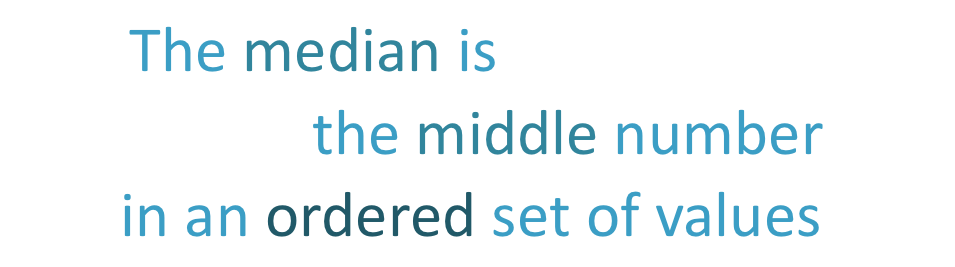The first step to find the median is to put the numbers in order from smallest to largest.

It is important to make sure that we have the same amount of numbers in the rewritten, ordered list as we did in the original list.The list of “6, 3, 1, 7, 4, 9” becomes “1, 3, 4, 6, 7, 9”.

Now that the numbers are in order, starting with the smallest and ending with the largest, we can find the middle value.

To find the middle value, we cross off the same amount of values at the beginning and end of our list of numbers.

We can cross off 2 numbers at the beginning of our list: 1 and 3.

And we can also cross off 2 numbers at the end of our list: 7 and 9.

We need to cross off the same amount of numbers at each end.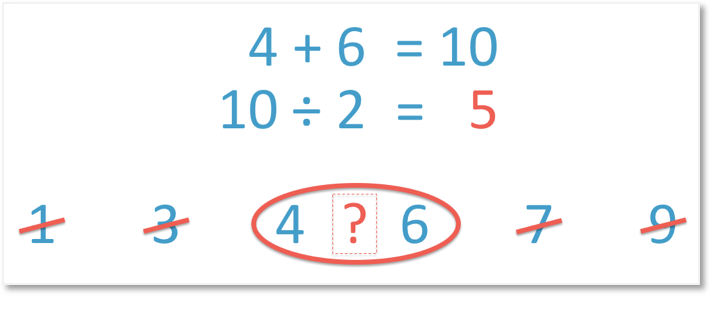There are two numbers remaining in the centre: 4 and 6.

If we crossed off another number at each end we would end up crossing out both the 4 and the 6 and all of the numbers would be crossed off.

Therefore when finding the median from an even amount of numbers, we will always stop crossing off numbers to leave two numbers remaining in the middle.

To find the median from these two remaining numbers, we need to find the number that is halfway between 4 and 6.

5 is directly between 4 and 6 and therefore 5 is our median value.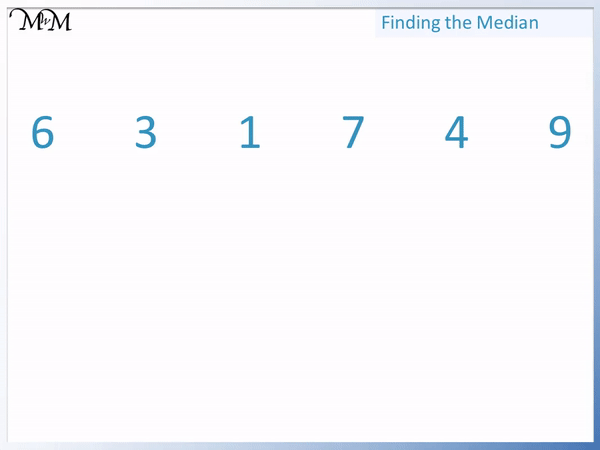It was easy to see that 5 was directly between 4 and 6.

However if the numbers are more spread apart, the median is not as obvious. <> There is an alternate method to find which value is in the middle of two numbers:

2. Divide this result by two.

For example, to find the number in between 4 and 6, we add 4 and 6 to get 10 and then divide 10 by 2 to get 5.

5 is directly between 4 and 6 and is the median of the list.

## Teaching Finding the Median with an Even Set

Here is another example of finding the median from an even set of numbers.

When teaching finding the median, it is important to remember to put the list of numbers in order from smallest to largest.

This step is commonly forgotten and it is the first thing to check if you are not arriving at the correct answer.

In this example, the list “8, 7, 6, 7, 3, 9, 0, 4” becomes “0, 3, 4, 6, 7, 7, 8, 9”.

After reordering the list, it is worth checking that there are the same amount of numbers in each list. If we have missed out a number, then the answer will be wrong.

We check that there are 8 numbers in total in each list.When there is an even amount of numbers, we cross off numbers at each end to leave two numbers in the middle.

We will always leave two numbers in the middle.

We have 0, 3, 4, 6 , 7, 7, 8, 9.

We have two numbers remaining: 6 and 7.

Because 6 and 7 are

, it is easy to find the halfway value.

6.5 is halfway between 6 and 7 and so, 6.5 is the median.

We simply put a the .5 after the smaller number. 6.5 is 6 and a half.

Alternatively, we can add 6 and 7 and then divide this by 2.

6 plus 7 equals 13 and half of 13 is 6.5.Now try our lesson on Finding the Mode where we learn what the mode of a list of numbers is.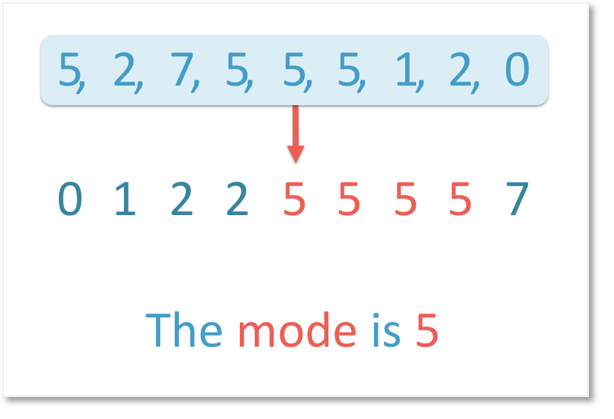error: Content is protected !!